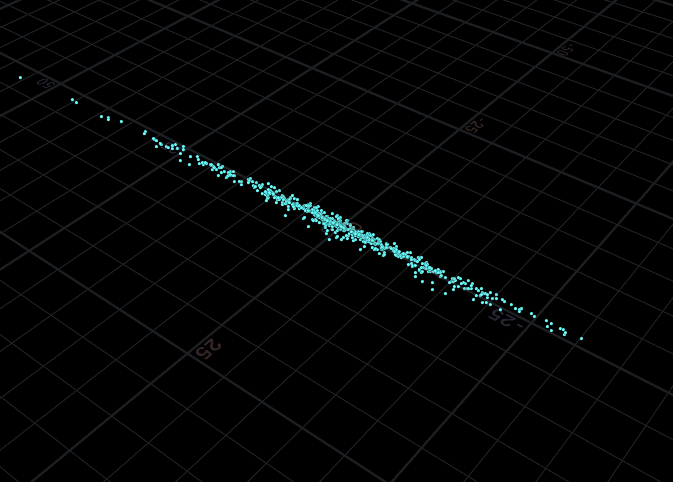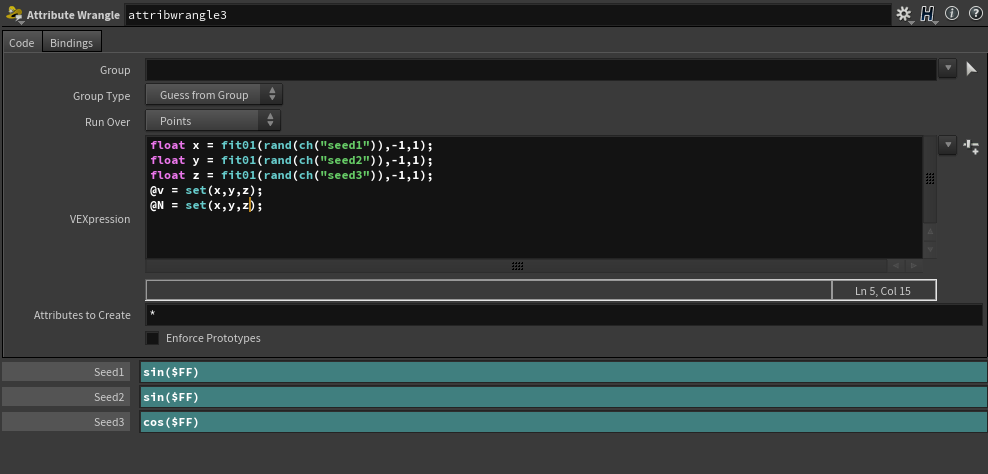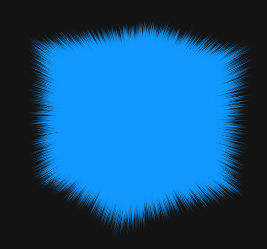# random normal direction

## Recommended Posts

I'm trying to control the normal of a single point. I want to use normals to visualize vectors that i will convert to velocity later. I've reduced my experiment to a single point to hopefully simplify things. I'm trying to make the normal point in a truly random direction for each frame. I tried this:

```float x = fit01(rand(ch("seed1")),-1,1);
float y = fit01(rand(ch("seed2")),-1,1);
float z = fit01(rand(ch("seed3")),-1,1);
@N = set(x,y,z);```

which gave me a random direction with in a disk. If i substitute @N for @v and feed this into a popnet that uses inherited vel i get particles in a plane. see attached image.

If I control each component of the vector leaving two at 0 and setting the third between -1 and 1 i can point the normal in any direction. I can't figure out how to get houdini to randomly set the normals direction in all directions.

Help appreciated.##### Share on other sites

I always do this with a one-liner like this:

`v@N = (vector(rand(ch("seed1")))-{0.5,0.5,0.5})*2;`

-James

•3
##### Share on other sites

here's my deconstruction...(of the orig setup)

Seeds.hipnc

•1
##### Share on other sites

Bonassus, I am no expert, but my explanation:

- casting vector() is there to get vector from float seed... because vex functions care about casting, see the long list: http://www.sidefx.com/docs/houdini/vex/functions/rand
- If you wish a uniform (x=y=z) scale from vector seed, use the `float()`

```vector        pos = 1;
float        seed = 0;

pos *= float(rand(seed));```

- and vice versa, Kappy gave float seed and did cast vector()

Btw Javier did a great tutorial here: https://vimeo.com/226167508

•1
##### Share on other sites

Vusta, thank you for the demonstration. I see you can get a roughly even distribution of directions by offsetting the sin cos functions. weird.

##### Share on other sites

James, thanks again for the vex solution.

v@v = (vector(rand(ch("seed1")))-{0.5,0.5,0.5})*2;

allow me to attempt an explanation. The rand function returns a value between 0 and 1. casting it as a vector creates a 3 float vector with 3 identical values. subtracting the 0.5 vector makes some values negative and then multiplying by 2 normalizes the values.

shouldn't this create a vector with three identical values? why is this equivalent to Noobini's offset solution?

##### Share on other sites

this confuses me at first too...until I read more carefully...here's the crucial part from help link above:

`If the result is a vector2, vector, or vector4, each component will be a different random number. `

so yeah as soon as you cast it as a vector (3 components) the seed is actually 3 different 'seeds'...as I manually offset in mine...

Edited by Noobini
##### Share on other sites

Randomly set the normals direction in all directions:

`@N = sample_direction_uniform(rand(@ptnum));`

3 hours ago, bonassus said:

allow me to attempt an explanation. The rand function returns a value between 0 and 1. casting it as a vector creates a 3 float vector with 3 identical values.

The rand() function implemented in many flavors: function signatures. You can do the same:

```float make_four()
{
return 4;
}

vector make_four()
{
return {4, 4, 4};
}```

Usually, VEX can guess which to use, saving your time. Sometimes it can't decide. "Ambiguous call to function ..." error may be raised, or unwanted value may be returned and converted silently. Like you said, if you need a random vector, but got vector with same random value copied across components. Use function-style cast to help VEX decide which function to use.

3 hours ago, bonassus said:

subtracting the 0.5 vector makes some values negative and then multiplying by 2 normalizes the values

Subtracting 0.5 offsets 0..1 range to -0.5..0.5, multiplying by 2 scales -0.5..0.5 range to -1..1. It is equivalent to fit01(value, -1, 1). For 3-float vectors everything repeated three times. Note that uniformly distributed random values in -1..1 range is a cube. You may want to normalize it to get normals of unit length:It wouldn't be uniformly distributed (corners of former cube will be more dense), but will work in most cases. To get uniform distribution, use sample_direction_uniform() function.

Edited by f1480187
"sample_sphere_uniform" should be "sample_direction_uniform"
##### Share on other sites

2 hours ago, Noobini said:

this confuses me at first too...until I read more carefully...here's the crucial part from help link above:

```
If the result is a vector2, vector, or vector4, each component will be a different random number. ```

so yeah as soon as you cast it as a vector (3 components) the seed is actually 3 different 'seeds'...as I manually offset in mine...

Oh okay. the rand function run once for each component in the vector() I assumed it was just casting the resulting value. thank you noobini.

F1, thanks for the clarification and the two examples that also do the same thing.

## Join the conversation

You can post now and register later. If you have an account, sign in now to post with your account.
Note: Your post will require moderator approval before it will be visible.×   Pasted as rich text.   Paste as plain text instead

Only 75 emoji are allowed.

×   Your previous content has been restored.   Clear editor

×   You cannot paste images directly. Upload or insert images from URL.

×
×